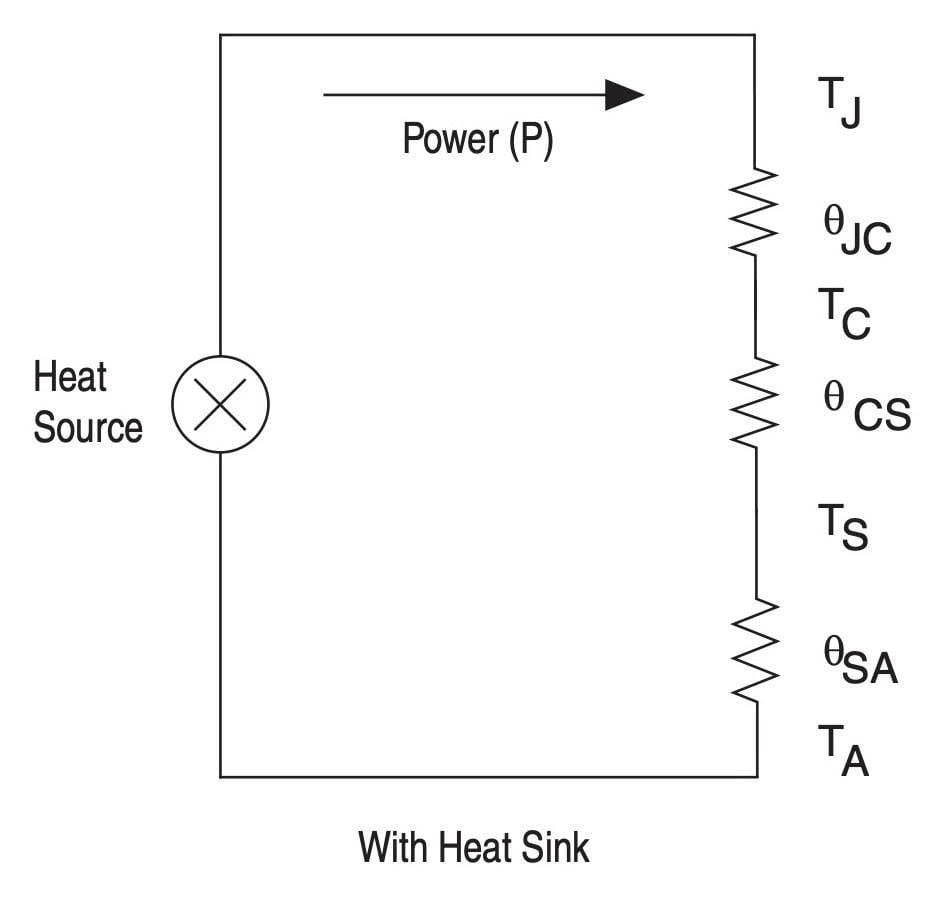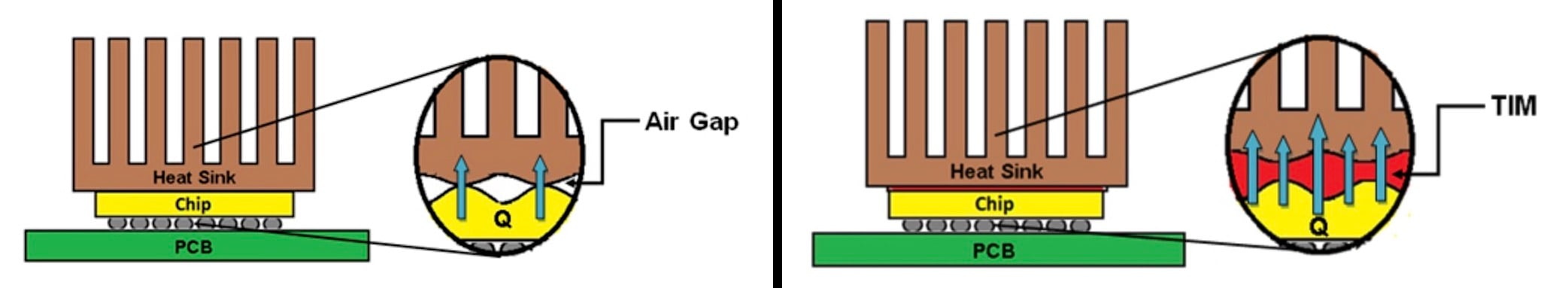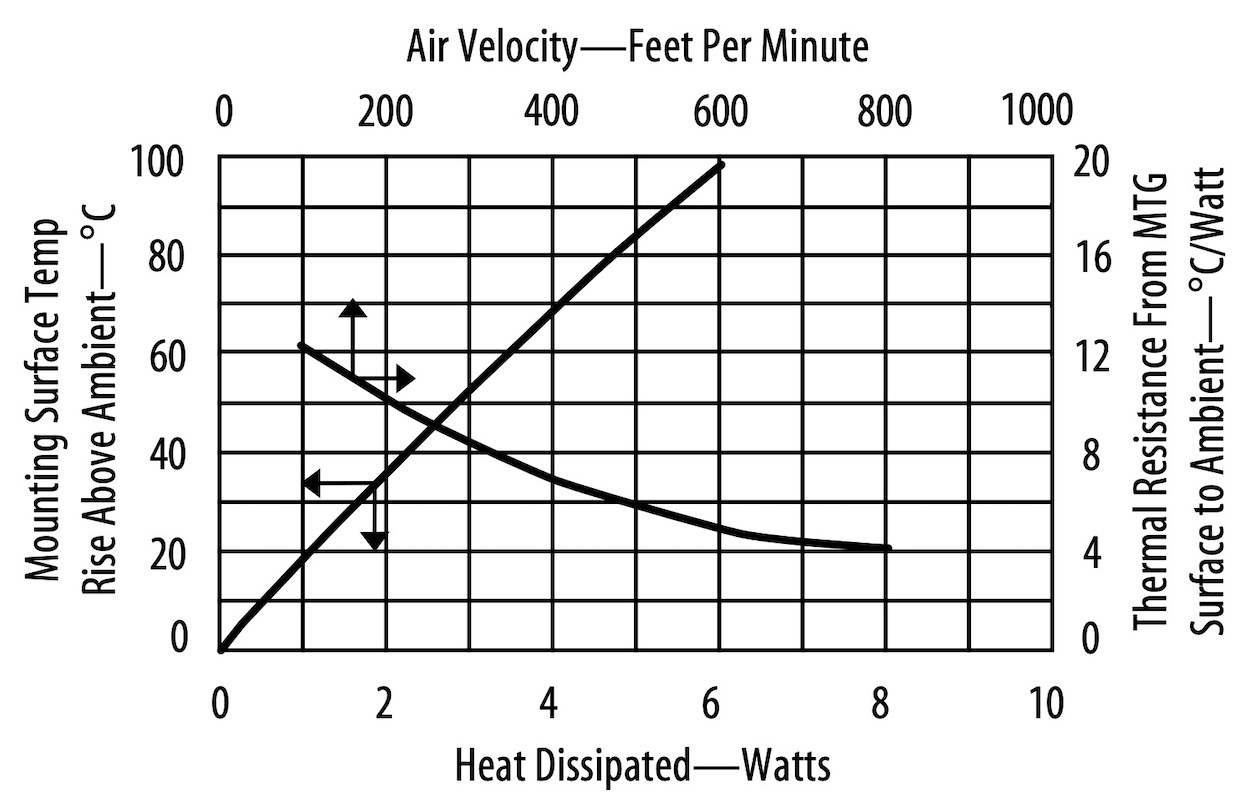As an Amazon Associate I earn from qualifying purchases

# Designing with a Heat Sink for Junction-to-Case Thermal Resistance

In the previous articles, we’ve discussed factors that affect the thermal performance of an IC package, including junction-to-ambient thermal resistance JA) (including optimal test conditions for θJA) and junction-to-case thermal resistance (θJC)

In this article, we’ll discuss thermal design differences for θJC when there is a heatsink present.

### Thermal Performance of a Design With a Heat Sink

If we’re given θJC, we can evaluate the thermal performance of a package attached to a heat sink. With an efficient heat sink, the heat flow path will consist of three thermal resistances in series as shown below:##### A thermal circuit model with a heat sink. Image used courtesy of Intel.

Here, θCS is the case-to-heatsink thermal resistance and θSA is the heatsink-to-ambient thermal resistance. The overall junction-to-ambient thermal resistance (θJA) of the system is given by:

\$\$θ_{JA,system} = θ_{JC} + θ_{CS} + θ_{SA}\$\$

##### Equation 2

θCS determines how easily the heat can flow from the package surface to the base of the heat sink. Due to the unevenness and irregularities of the package and heatsink surfaces, an air gap can be formed between the two surfaces. This air gap represents significant resistance to heat flow and should be avoided. To remove this air gap, a thermal interface material (TIM) is inserted in between the two surfaces.##### A comparison of the interface between a heat sink and an IC without (left) and with (right) TIM. Adapted image used courtesy of TTS Group

TIMs are heat conductive materials that can enhance the thermal coupling between the heat sink and the package. θCS is a function of the TIM thermal conductivity, its thickness, and the area over which it is spread. The following equation can be used to calculate θCS from the TIM properties:

\$\$θ_{CS} = (frac{Thickness}{Area}) times frac{1}{Conductivity}\$\$

θSA specifies the thermal resistance from the base of the heat sink to the ambient environment. This can be found in the heat sink datasheet. For example, the graph below shows that Aavid ML26AAG has a thermal resistance of 10°C/W at 200 feet per minute air velocity.#### Example

Assume that the datasheet thermal data of a particular IC is:

θJA = 16°C/W

θJC = 3°C/W

Suppose that the ambient temperature and the maximum junction temperature are 50°C and 120°C, respectively. And, the IC burns 10 W of power. Do we need a heat sink for this application?

In order to determine whether a heat sink is required or not, we can use the θJA parameter provided by the datasheet:

\$\$T_J = T_A + P times θ_{JA} = 50 + 10 times 16 = 210°C\$\$

This exceeds the intended maximum junction temperature (120°C). Hence, a heat sink is required. It should be noted that, as discussed in a previous article, manufacturers measure the θJA parameter under specific test conditions that can be very different from our end design and applying datasheet θJA to an application-specific board might not give us an accurate estimation of the design thermal performance. However, technical documents from several different manufacturers, such as Intel and Microsemi, use this thermal metric to obtain a first-order estimation of the junction temperature and decide whether a heat sink is required or not.

What is the maximum permissible thermal resistance for the heat sink? Having TJ and TA, we can calculate the maximum junction-to-ambient thermal resistance θJA,max for our design:

\$\$θ_{JA,max} = frac{T_J – T_A}{P} = frac{120 – 50}{10} = 7°C/W\$\$

Substituting θJC = 3 °C/W and θJA,max = 7 °C/W in Equation 2 gives:

\$\$θ_{CS} + θ_{SA} = 4°C/W\$\$

Assuming that a TIM with a thermal resistance of θCS = 0.4 °C/W is used, we obtain:

\$\$θ_{SA} = 3.6°C/W\$\$

Hence, a heat sink with a thermal resistance of 3.6 °C/W or better should be employed.

### What About a Design Without a Heatsink?

For designs without a heatsink, different technical documents usually give the following equation:

\$\$θ_{JA,system} = θ_{JC} + θ_{CA}\$\$

where θCA is the case-to-ambient thermal resistance. This equation relates the package θJC to the system thermal resistance θJA,system.

This can be actually valid for a device with a metal package where the package temperature is almost constant and there is negligible thermal coupling between the package and the PC board. However, with today’s plastic or ceramic packages, there is significant coupling between the package and the board and a large temperature gradient can be observed on the package. This makes θCA a function of the board properties and the above equation questionable.

Hence, it seems that, in practice, there is not a straightforward way to use the above equation for evaluating the thermal performance of a design. In the next article in this series, we’ll look at two other commonly used thermal metrics: ΨJT and ΨJB. We’ll see that these can give us a more accurate estimation of the junction temperature in an application-specific environment.